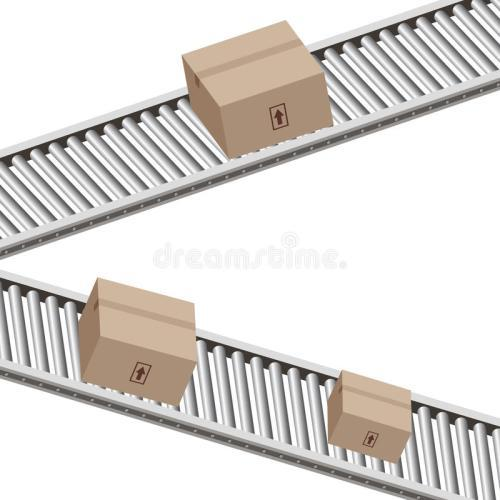# Conveyor Belt Cost

- Jan 02, 2019-

The role of the conveyor belt in practical applications is that there are many kinds of conveyor belts that can be continuous, high efficiency, large angle of inclination transportation, safe operation of the conveyor belt, easy to use conveyor belt, easy maintenance, low freight, and can shorten the transportation distance and reduce The project cost, saving manpower and material resources, is widely used in many fields, but how about the price of conveyor belts? How much is a conveyor belt?Conveyor belt pricing unit

Layered conveyor belt: yuan / standard square meter steel cord conveyor belt: yuan / standard square meters

PVC and PVG whole core flame retardant conveyor belt: yuan / natural square meterConveyor belt price calculation method

Standard square meters = bandwidth (m) × (number of layers + (overlay thickness + under cover rubber thickness) / 1.5) × belt length (m)

Steel cord conveyor belt:

Standard square meters = bandwidth (m) × product thickness (mm) / 1.5 × belt length (m) The calculation of the natural square meter of PVC and PVG whole core flame retardant conveyor belt is the bandwidth × belt length. Note the length of the conveyor belt. It refers to the length of the conveyor belt when it is flattened, and is generally marked by the manufacturer at the time of production.How much is a typical conveyor belt? The manufacturer's conveyor belt calculation method looks at the first one: The price of the layered belt conveyor is generally calculated by the square. The formula: the square of one meter belt = the bandwidth m * [(the thickness of the glue is mm + the thickness of the glue is mm) / 1.5+ cloth layer number, for example, CC 800*6 (4.5+1.5) ordinary polyester-cotton conveyor belt, CC is made of cotton canvas, 800 is the width of the belt is 800mm, 6 is the number of layers, 4.5+1.5 are respectively The thickness of the glue and the glue, if the unit price is 25 yuan / square, then the price per meter is calculated according to the formula: 25 * 8 = 200 yuan / meter.

The second type: fabric whole core flame retardant conveyor belt is unit price * bandwidth * belt length

The third type: steel cord conveyor belt price manufacturers are generally calculated by square, formula: square meter of one meter belt = bandwidth m * (gluing thickness mm + rubber thickness mm + wire rope diameter mm) / 1.5, data in parentheses in the country There are regulations in the standard. For example, the price of ST1250 B1200mm, st represents steel wire, 1250 is the tensile strength of the whole belt, 1200 is the bandwidth of 1200mm, the standard specifies that the thickness of the tape is 6mm, the thickness of the rubber is 6mm, the diameter of the wire rope is 4.5mm, if the unit price is 45 yuan / square, then calculate the price per meter according to the formula: 45 * 11 = 495 yuan / meter.